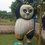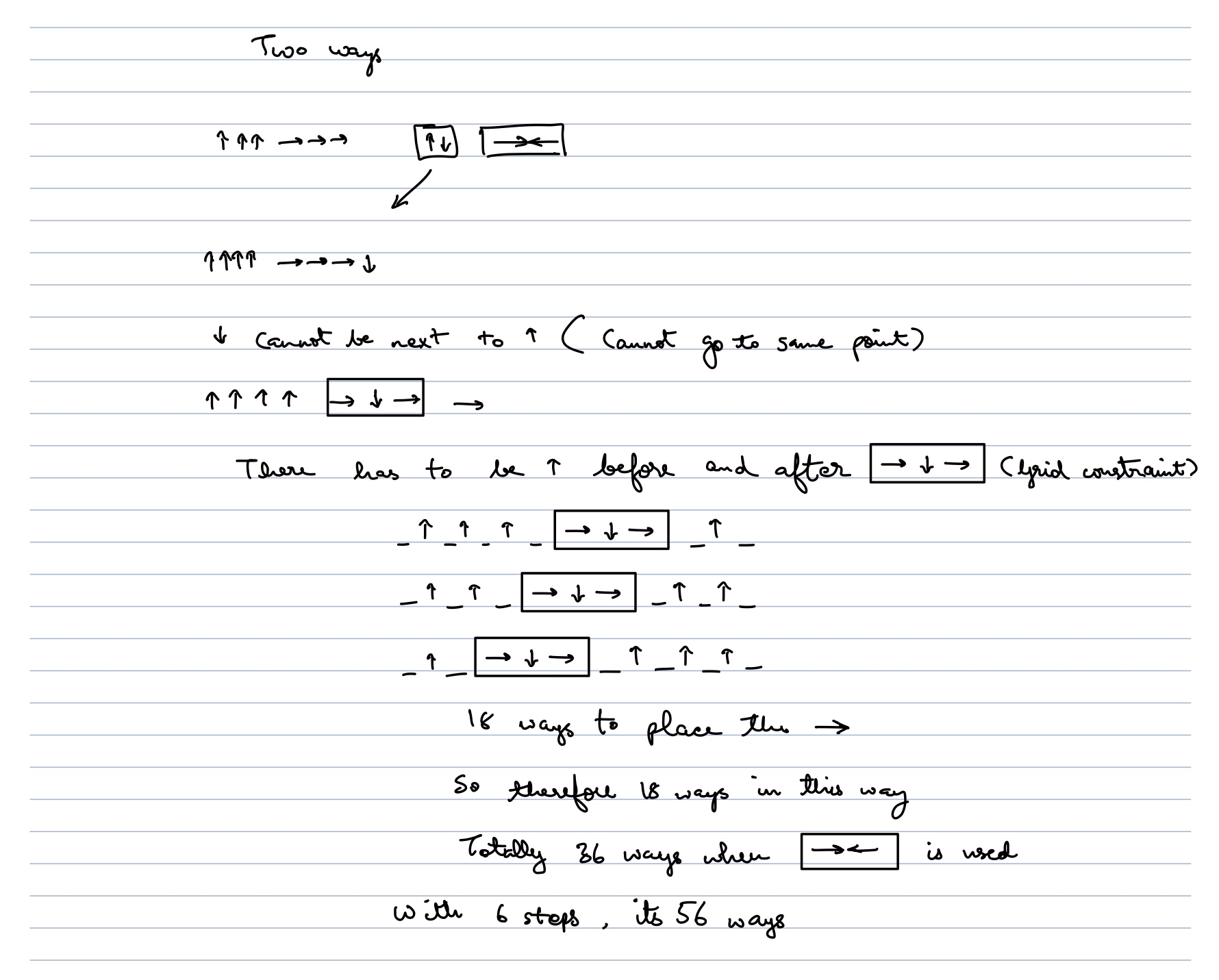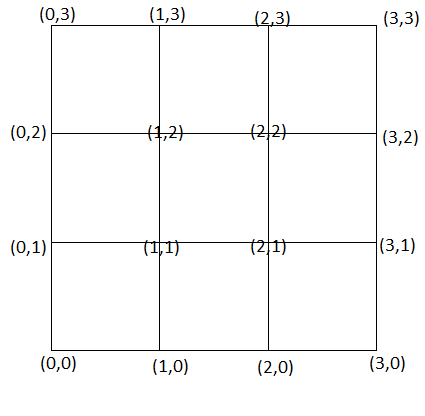# Help: Grid Walking

In a $3$x$3$ grid composed of $4$ vertical lines and $4$ horizontal lines; a beetle is moving from one intersecting point to another in one step. If the beetle moves from the bottom left corner to the top right corner within $8$ steps, and it never returned to the same intersecting point, what is the number of different paths the beetle can take?Note by Ryan Merino
4 months, 1 week ago

This discussion board is a place to discuss our Daily Challenges and the math and science related to those challenges. Explanations are more than just a solution — they should explain the steps and thinking strategies that you used to obtain the solution. Comments should further the discussion of math and science.

When posting on Brilliant:

• Use the emojis to react to an explanation, whether you're congratulating a job well done , or just really confused .
• Ask specific questions about the challenge or the steps in somebody's explanation. Well-posed questions can add a lot to the discussion, but posting "I don't understand!" doesn't help anyone.
• Try to contribute something new to the discussion, whether it is an extension, generalization or other idea related to the challenge.

MarkdownAppears as
*italics* or _italics_ italics
**bold** or __bold__ bold
- bulleted- list
• bulleted
• list
1. numbered2. list
1. numbered
2. list
Note: you must add a full line of space before and after lists for them to show up correctly
paragraph 1paragraph 2

paragraph 1

paragraph 2

[example link](https://brilliant.org)example link
> This is a quote
This is a quote
    # I indented these lines
# 4 spaces, and now they show
# up as a code block.

print "hello world"
# I indented these lines
# 4 spaces, and now they show
# up as a code block.

print "hello world"
MathAppears as
Remember to wrap math in $$ ... $$ or $ ... $ to ensure proper formatting.
2 \times 3 $2 \times 3$
2^{34} $2^{34}$
a_{i-1} $a_{i-1}$
\frac{2}{3} $\frac{2}{3}$
\sqrt{2} $\sqrt{2}$
\sum_{i=1}^3 $\sum_{i=1}^3$
\sin \theta $\sin \theta$
\boxed{123} $\boxed{123}$

Sort by:

@Zakir Husain I got 36 for exactly 8 steps, I think I might have over counted, is there any mistake you see in my approach?- 4 months, 1 week ago

There are total $36$ paths I found it using a Python program

- 4 months, 1 week ago

That I think would be for exactly 8 steps, including 6 steps will make it 36

- 4 months, 1 week ago$Following\space are\space sequence\space of\space movements:$ $(0, 0)\to (1, 0)\to (2, 0)\to (3, 0)\to (3, 1)\to (3, 2)\to (2, 2)\to (2, 3)\to (3, 3)$ $(0, 0)\to (1, 0)\to (2, 0)\to (3, 0)\to (3, 1)\to (2, 1)\to (2, 2)\to (3, 2)\to (3, 3)$ $(0, 0)\to (1, 0)\to (2, 0)\to (3, 0)\to (3, 1)\to (2, 1)\to (2, 2)\to (2, 3)\to (3, 3)$ $(0, 0)\to (1, 0)\to (2, 0)\to (2, 1)\to (3, 1)\to (3, 2)\to (2, 2)\to (2, 3)\to (3, 3)$ $(0, 0)\to (1, 0)\to (2, 0)\to (2, 1)\to (2, 2)\to (1, 2)\to (1, 3)\to (2, 3)\to (3, 3)$ $(0, 0)\to (1, 0)\to (2, 0)\to (2, 1)\to (1, 1)\to (1, 2)\to (2, 2)\to (3, 2)\to (3, 3)$ $(0, 0)\to (1, 0)\to (2, 0)\to (2, 1)\to (1, 1)\to (1, 2)\to (2, 2)\to (2, 3)\to (3, 3)$ $(0, 0)\to (1, 0)\to (2, 0)\to (2, 1)\to (1, 1)\to (1, 2)\to (1, 3)\to (2, 3)\to (3, 3)$ $(0, 0)\to (1, 0)\to (1, 1)\to (2, 1)\to (3, 1)\to (3, 2)\to (2, 2)\to (2, 3)\to (3, 3)$ $(0, 0)\to (1, 0)\to (1, 1)\to (2, 1)\to (2, 2)\to (1, 2)\to (1, 3)\to (2, 3)\to (3, 3)$ $(0, 0)\to (1, 0)\to (1, 1)\to (2, 1)\to (2, 0)\to (3, 0)\to (3, 1)\to (3, 2)\to (3, 3)$ $(0, 0)\to (1, 0)\to (1, 1)\to (1, 2)\to (2, 2)\to (2, 1)\to (3, 1)\to (3, 2)\to (3, 3)$ $(0, 0)\to (1, 0)\to (1, 1)\to (1, 2)\to (1, 3)\to (2, 3)\to (2, 2)\to (3, 2)\to (3, 3)$ $(0, 0)\to (1, 0)\to (1, 1)\to (1, 2)\to (0, 2)\to (0, 3)\to (1, 3)\to (2, 3)\to (3, 3)$ $(0, 0)\to (1, 0)\to (1, 1)\to (0, 1)\to (0, 2)\to (1, 2)\to (2, 2)\to (3, 2)\to (3, 3)$ $(0, 0)\to (1, 0)\to (1, 1)\to (0, 1)\to (0, 2)\to (1, 2)\to (2, 2)\to (2, 3)\to (3, 3)$ $(0, 0)\to (1, 0)\to (1, 1)\to (0, 1)\to (0, 2)\to (1, 2)\to (1, 3)\to (2, 3)\to (3, 3)$ $(0, 0)\to (1, 0)\to (1, 1)\to (0, 1)\to (0, 2)\to (0, 3)\to (1, 3)\to (2, 3)\to (3, 3)$ $(0, 0)\to (0, 1)\to (1, 1)\to (2, 1)\to (3, 1)\to (3, 2)\to (2, 2)\to (2, 3)\to (3, 3)$ $(0, 0)\to (0, 1)\to (1, 1)\to (2, 1)\to (2, 2)\to (1, 2)\to (1, 3)\to (2, 3)\to (3, 3)$ $(0, 0)\to (0, 1)\to (1, 1)\to (2, 1)\to (2, 0)\to (3, 0)\to (3, 1)\to (3, 2)\to (3, 3)$ $(0, 0)\to (0, 1)\to (1, 1)\to (1, 2)\to (2, 2)\to (2, 1)\to (3, 1)\to (3, 2)\to (3, 3)$ $(0, 0)\to (0, 1)\to (1, 1)\to (1, 2)\to (1, 3)\to (2, 3)\to (2, 2)\to (3, 2)\to (3, 3)$ $(0, 0)\to (0, 1)\to (1, 1)\to (1, 2)\to (0, 2)\to (0, 3)\to (1, 3)\to (2, 3)\to (3, 3)$ $(0, 0)\to (0, 1)\to (1, 1)\to (1, 0)\to (2, 0)\to (3, 0)\to (3, 1)\to (3, 2)\to (3, 3)$ $(0, 0)\to (0, 1)\to (1, 1)\to (1, 0)\to (2, 0)\to (2, 1)\to (3, 1)\to (3, 2)\to (3, 3)$ $(0, 0)\to (0, 1)\to (1, 1)\to (1, 0)\to (2, 0)\to (2, 1)\to (2, 2)\to (3, 2)\to (3, 3)$ $(0, 0)\to (0, 1)\to (1, 1)\to (1, 0)\to (2, 0)\to (2, 1)\to (2, 2)\to (2, 3)\to (3, 3)$ $(0, 0)\to (0, 1)\to (0, 2)\to (1, 2)\to (2, 2)\to (2, 1)\to (3, 1)\to (3, 2)\to (3, 3)$ $(0, 0)\to (0, 1)\to (0, 2)\to (1, 2)\to (1, 3)\to (2, 3)\to (2, 2)\to (3, 2)\to (3, 3)$ $(0, 0)\to (0, 1)\to (0, 2)\to (1, 2)\to (1, 1)\to (2, 1)\to (3, 1)\to (3, 2)\to (3, 3)$ $(0, 0)\to (0, 1)\to (0, 2)\to (1, 2)\to (1, 1)\to (2, 1)\to (2, 2)\to (3, 2)\to (3, 3)$ $(0, 0)\to (0, 1)\to (0, 2)\to (1, 2)\to (1, 1)\to (2, 1)\to (2, 2)\to (2, 3)\to (3, 3)$ $(0, 0)\to (0, 1)\to (0, 2)\to (0, 3)\to (1, 3)\to (2, 3)\to (2, 2)\to (3, 2)\to (3, 3)$ $(0, 0)\to (0, 1)\to (0, 2)\to (0, 3)\to (1, 3)\to (1, 2)\to (2, 2)\to (3, 2)\to (3, 3)$ $(0, 0)\to (0, 1)\to (0, 2)\to (0, 3)\to (1, 3)\to (1, 2)\to (2, 2)\to (2, 3)\to (3, 3)$

- 4 months, 1 week ago

$(0,0)→(0,1)→(0,0)→(1,0)→(1,1)→(1,2)→(1,3)→(2,3)→(3,3)$

This path isn’t allowed because we come back to origin again, I think so without these ones there should be 36 paths with length 8

- 4 months, 1 week ago

OIC found new mistake ;)

- 4 months, 1 week ago

Edited!

- 4 months, 1 week ago

I don’t see any path like this

$(0,0) ➝ (0,1) ➝ (1,1) ➝ …$

One example of a complete path not present

$(0,0) ➝ (0,1) ➝ (1,1) ➝ (1,2) ➝ (2,2) ➝ (2,1) ➝ (3,1) ➝ (3,2) ➝ (3,3)$

(I do silly mistakes all the time, I might have not understood the question too)

- 4 months, 1 week ago

There was a problem in my program, I fixed it and found $56$ paths

- 4 months, 1 week ago

@Jeff Giff Now I edited My comment

- 4 months, 1 week ago